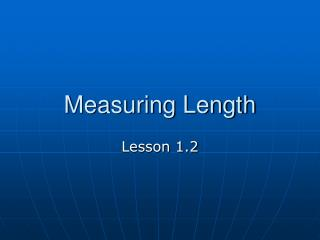DownloadDownload PresentationMeasuring Length

# Measuring Length

Télécharger la présentation## Measuring Length

- - - - - - - - - - - - - - - - - - - - - - - - - - - E N D - - - - - - - - - - - - - - - - - - - - - - - - - - -
##### Presentation Transcript

1. Measuring Length Lesson 1.2

2. A trip down memory lane… -5 -4 -3 -2 -1 0 1 2 3 4 5 6 The number line

3. Any point on the number line corresponds to only one number A B -5 -4 -3 -2 -1 0 1 2 3 4 5 6 Point A is at -3 Point B is at 4 The coordinate of a point on the number line is a real number. In the illustration, -3 is the coordinate of point A and 4 is the coordinate of point B.

4. Length of a segment AB m AB AB A B -5 -4 -3 -2 -1 0 1 2 3 4 5 6 The length of a segment is the number of spaces between the endpoints on the number line. The length of segment AB in this case is 7 Another way to find the length of segment AB is to find the positive difference between the two coordinates. 4 – (-3) = 7 or (-3) – 4 = -7 Thus, the length of segment AB is 7. (ALWAYS POSITIVE)

5. If point A is on -2, point B is on 6, and point X is on 2, then find the length of the following. AB = AX = XB = A X B -5 -4 -3 -2 -1 0 1 2 3 4 5 6 8 4 What is the relationship between AX and XB? 4

6. Definition of congruent Congruent means having the same measure. Congruent means almost the same thing as equal.

7. Congruent segments A B C D This says “segment AB is congruent to segment CD” It means they have the same length

8. Segment Congruence Postulate • If two segments have the same length, then the segments are congruent. Also if two segments are congruent, then they have the same length. • 1.2.2

9. How to mark congruent segments in figures A B C F G D E

10. Segment addition postulate If point R is between points P and Q on a line, then PR + RQ = PQ (1.2.3) P R Q

11. Point R is between points P and Q on a line. 1. PR = 13 RQ = 8 PQ = 2. PR = RQ = 11 PQ = 33 3. PR = 12 RQ = PQ = 22 P R Q 22 10 21

12. Point R is between points P and Q on a line. 1. PR = 3x + 1 RQ = x + 4 PQ = 25 x = 2. PR = 5x PR = 7x - 8 x = P R Q 4 5

13. Assignment • A3 • pp. 21-22 #1, 3, 6-9, 11-25